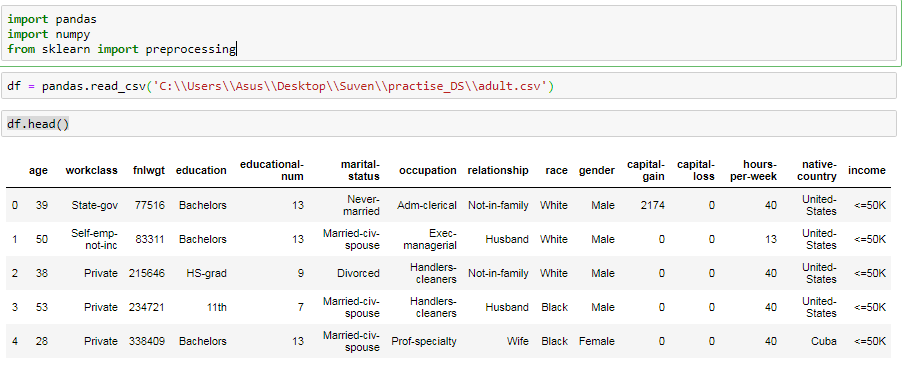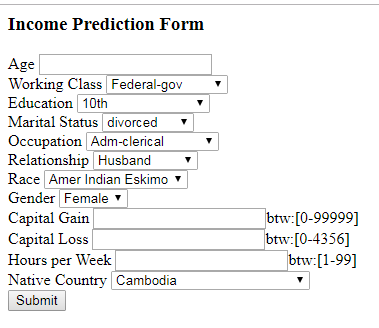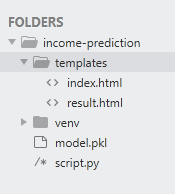Related Articles
Deploy Machine Learning Model using Flask
• Difficulty Level : Easy
• Last Updated : 03 Oct, 2019

Machine learning is a process which is widely used for prediction. N number of algorithms are available in various libraries which can be used for prediction. In this article, we are going to build a prediction model on historic data using different machine learning algorithms and classifiers, plot the results and calculate the accuracy of the model on the testing data.
Building/Training a model using various algorithms on a large dataset is one part of the data. But using these model within different application is second part of deploying machine learning in the real world.

To put it to use in order to predict the new data, we have to deploy it over the internet so that the outside world can use it. In this article, we will talk about how we have trained a machine learning model, created a web application on it using Flask.

We have to install many required libraries which will be used in this model. Use pip command to install all the libraries.

```pip install pandas
pip install numpy
pip install sklearn```

Decision Tree –
Decision Tree is a well known supervised machine learning algorithm because its ease to use, resilient and flexible. I have implemented the algorithm on Adult dataset from UCI machine learning repository.

Get the data –
You can get the dataset from this link.

Getting the dataset is not the end. We have to preprocess the data, means we need to clean the dataset. Cleaning of the dataset includes different types of process like remove missing values, fill NA values etc.

 `# importing the dataset ` `import` `pandas ` `import` `numpy ` `from` `sklearn ``import` `preprocessing ` ` `  `df ``=` `pandas.read_csv(``'adult.csv'``)     ` `df.head() `

Output :Preprocessing the dataset –
It consists of 14 attributes and a class label telling whether the income of the individual is less than or more than 50K a year. These attributes range from the age of the person, the working class label to relationship status and the race the person belongs to. The information about all the attributes can be found here.

At first, we find and remove any missing values from the data. We have replaced the missing values with the mode value in that column. There are many other ways to replace missing values but for this type of dataset it seemed most optimal.

 `df ``=` `df.drop([``'fnlwgt'``, ``'educational-num'``], axis ``=` `1``) ` ` `  `col_names ``=` `df.columns ` ` `  `for` `c ``in` `col_names: ` `    ``df ``=` `df.replace(``"?"``, numpy.NaN) ` `df ``=` `df.``apply``(``lambda` `x:x.fillna(x.value_counts().index[``0``])) `

The machine learning algorithm cannot process categorical data values. It can only process numerical values.
To fit the data into the prediction model, we need to convert categorical values to numerical ones. Before that, we will evaluate if any transformation on categorical columns is necessary.

Discretization – It is a common way to make categorical data more tidy and meaningful. We have applied discretization on column `marital_status `where they are narrowed down to only to values married or not married. Later, we will apply label encoder in the remaining data columns. Also, there are two redundant columns `{‘education’, ‘educational-num’}`, therefore, we have removed one of them.

 `df.replace([``'Divorced'``, ``'Married-AF-spouse'``,  ` `              ``'Married-civ-spouse'``, ``'Married-spouse-absent'``,  ` `              ``'Never-married'``, ``'Separated'``, ``'Widowed'``], ` `             ``[``'divorced'``, ``'married'``, ``'married'``, ``'married'``, ` `              ``'not married'``, ``'not married'``, ``'not married'``], inplace ``=` `True``) ` ` `  `category_col ``=``[``'workclass'``, ``'race'``, ``'education'``, ``'marital-status'``, ``'occupation'``, ` `               ``'relationship'``, ``'gender'``, ``'native-country'``, ``'income'``]  ` `labelEncoder ``=` `preprocessing.LabelEncoder() ` ` `  `mapping_dict ``=``{} ` `for` `col ``in` `category_col: ` `    ``df[col] ``=` `labelEncoder.fit_transform(df[col]) ` ` `  `    ``le_name_mapping ``=` `dict``(``zip``(labelEncoder.classes_, ` `                        ``labelEncoder.transform(labelEncoder.classes_))) ` ` `  `    ``mapping_dict[col]``=` `le_name_mapping ` `print``(mapping_dict) `

Output :

{‘workclass’: {‘ ?’: 0, ‘ Federal-gov’: 1, ‘ Local-gov’: 2, ‘ Never-worked’: 3, ‘ Private’: 4, ‘ Self-emp-inc’: 5, ‘ Self-emp-not-inc’: 6, ‘ State-gov’: 7, ‘ Without-pay’: 8}, ‘race’: {‘ Amer-Indian-Eskimo’: 0, ‘ Asian-Pac-Islander’: 1, ‘ Black’: 2, ‘ Other’: 3, ‘ White’: 4}, ‘education’: {‘ 10th’: 0, ‘ 11th’: 1, ‘ 12th’: 2, ‘ 1st-4th’: 3, ‘ 5th-6th’: 4, ‘ 7th-8th’: 5, ‘ 9th’: 6, ‘ Assoc-acdm’: 7, ‘ Assoc-voc’: 8, ‘ Bachelors’: 9, ‘ Doctorate’: 10, ‘ HS-grad’: 11, ‘ Masters’: 12, ‘ Preschool’: 13, ‘ Prof-school’: 14, ‘ Some-college’: 15}, ‘marital-status’: {‘ Divorced’: 0, ‘ Married-AF-spouse’: 1, ‘ Married-civ-spouse’: 2, ‘ Married-spouse-absent’: 3, ‘ Never-married’: 4, ‘ Separated’: 5, ‘ Widowed’: 6}, ‘occupation’: {‘ ?’: 0, ‘ Adm-clerical’: 1, ‘ Armed-Forces’: 2, ‘ Craft-repair’: 3, ‘ Exec-managerial’: 4, ‘ Farming-fishing’: 5, ‘ Handlers-cleaners’: 6, ‘ Machine-op-inspct’: 7, ‘ Other-service’: 8, ‘ Priv-house-serv’: 9, ‘ Prof-specialty’: 10, ‘ Protective-serv’: 11, ‘ Sales’: 12, ‘ Tech-support’: 13, ‘ Transport-moving’: 14}, ‘relationship’: {‘ Husband’: 0, ‘ Not-in-family’: 1, ‘ Other-relative’: 2, ‘ Own-child’: 3, ‘ Unmarried’: 4, ‘ Wife’: 5}, ‘gender’: {‘ Female’: 0, ‘ Male’: 1}, ‘native-country’: {‘ ?’: 0, ‘ Cambodia’: 1, ‘ Canada’: 2, ‘ China’: 3, ‘ Columbia’: 4, ‘ Cuba’: 5, ‘ Dominican-Republic’: 6, ‘ Ecuador’: 7, ‘ El-Salvador’: 8, ‘ England’: 9, ‘ France’: 10, ‘ Germany’: 11, ‘ Greece’: 12, ‘ Guatemala’: 13, ‘ Haiti’: 14, ‘ Holand-Netherlands’: 15, ‘ Honduras’: 16, ‘ Hong’: 17, ‘ Hungary’: 18, ‘ India’: 19, ‘ Iran’: 20, ‘ Ireland’: 21, ‘ Italy’: 22, ‘ Jamaica’: 23, ‘ Japan’: 24, ‘ Laos’: 25, ‘ Mexico’: 26, ‘ Nicaragua’: 27, ‘ Outlying-US(Guam-USVI-etc)’: 28, ‘ Peru’: 29, ‘ Philippines’: 30, ‘ Poland’: 31, ‘ Portugal’: 32, ‘ Puerto-Rico’: 33, ‘ Scotland’: 34, ‘ South’: 35, ‘ Taiwan’: 36, ‘ Thailand’: 37, ‘ Trinadad&Tobago’: 38, ‘ United-States’: 39, ‘ Vietnam’: 40, ‘ Yugos lavia’: 41}, ‘income’: {‘ 50K’: 1}}

Fitting the model –
After pre-processing the data, the data is ready to feed it to the machine learning algorithm. We then slice the data separating the labels with the attributes. Now, we split the dataset into two halves, one for training and on for testing. This is achieved using `train_test_split()` function of sklearn.

 `from` `sklearn.model_selection ``import` `train_test_split ` `from` `sklearn.tree ``import` `DecisionTreeClassifier ` `from` `sklearn.metrics ``import` `accuracy_score ` ` `  `X ``=` `df.values[:, ``0``:``12``] ` `Y ``=` `df.values[:, ``12``] `

We have used decision tree classifier here as a predicting model. We fed the training part of the data to train the model.
Once training is done, we test what is the accuracy of the model by providing testing part of the data to the model.
With this, we achieve an accuracy of 84% approximately. Now in order to use this model with new unknown data, we need to save the model so that we can predict the values later. For this, we make use of pickle in Python which is a powerful algorithm for serializing and de-serializing a Python object structure.

 `X_train, X_test, y_train, y_test ``=` `train_test_split( ` `           ``X, Y, test_size ``=` `0.3``, random_state ``=` `100``) ` ` `  `dt_clf_gini ``=` `DecisionTreeClassifier(criterion ``=` `"gini"``, ` `                                     ``random_state ``=` `100``, ` `                                     ``max_depth ``=` `5``, ` `                                     ``min_samples_leaf ``=` `5``) ` ` `  `dt_clf_gini.fit(X_train, y_train) ` `y_pred_gini ``=` `dt_clf_gini.predict(X_test) ` ` `  `print` `(``"Desicion Tree using Gini Index\nAccuracy is "``, ` `             ``accuracy_score(y_test, y_pred_gini)``*``100` `) `

Output :

```Desicion Tree using Gini Index
Accuracy is  83.13031016480704
```

Flask is a Python-based microframework used for developing small scale websites. Flask is very easy to make Restful API’s using python. As of now, we have develop a model i.e `model.pkl` which can predict a class of the data based on a various attribute of the data. The class label is Salary >=50K or <50K.
Now we will design a web application where the user will input all the attribute values and the data will be given the model, based on the training given to the model, the model will predict the what should be the salary of the person whose details has been fed.

HTML Form –
For predicting the income from various attributes we first need to collect the data(new attribute values) and then use the decision tree model we build above to predict whether the income is more than 50K or less. Therefore, in order to collect the data we create html form which would contain all the different options to select from each attribute. Here, we have created a simple form using html only. If you want to make the form more interactive you can do so as well.

 `<``html``> ` `<``body``> ` `    ``<``h3``>Income Prediction Form ` ` `  `<``div``> ` `  ``<``form` `action``=``"/result"` `method``=``"POST"``> ` `    ``<``label` `for``=``"age"``>Age ` `    ``<``input` `type``=``"text"` `id``=``"age"` `name``=``"age"``> ` `    ``<``br``> ` `    ``<``label` `for``=``"w_class"``>Working Class ` `    ``<``select` `id``=``"w_class"` `name``=``"w_class"``> ` `      ``<``option` `value``=``"0"``>Federal-gov ` `      ``<``option` `value``=``"1"``>Local-gov ` `      ``<``option` `value``=``"2"``>Never-worked ` `      ``<``option` `value``=``"3"``>Private ` `      ``<``option` `value``=``"4"``>Self-emp-inc ` `      ``<``option` `value``=``"5"``>Self-emp-not-inc ` `      ``<``option` `value``=``"6"``>State-gov ` `      ``<``option` `value``=``"7"``>Without-pay ` `    `` ` `    ``<``br``> ` `    ``<``label` `for``=``"edu"``>Education ` `    ``<``select` `id``=``"edu"` `name``=``"edu"``> ` `      ``<``option` `value``=``"0"``>10th ` `      ``<``option` `value``=``"1"``>11th ` `      ``<``option` `value``=``"2"``>12th ` `      ``<``option` `value``=``"3"``>1st-4th ` `      ``<``option` `value``=``"4"``>5th-6th ` `      ``<``option` `value``=``"5"``>7th-8th ` `      ``<``option` `value``=``"6"``>9th ` `      ``<``option` `value``=``"7"``>Assoc-acdm ` `      ``<``option` `value``=``"8"``>Assoc-voc ` `      ``<``option` `value``=``"9"``>Bachelors ` `      ``<``option` `value``=``"10"``>Doctorate ` `      ``<``option` `value``=``"11"``>HS-grad ` `      ``<``option` `value``=``"12"``>Masters ` `      ``<``option` `value``=``"13"``>Preschool ` `      ``<``option` `value``=``"14"``>Prof-school ` `      ``<``option` `value``=``"15"``>16 - Some-college ` `    `` ` `    ``<``br``> ` `    ``<``label` `for``=``"martial_stat"``>Marital Status ` `    ``<``select` `id``=``"martial_stat"` `name``=``"martial_stat"``> ` `      ``<``option` `value``=``"0"``>divorced ` `      ``<``option` `value``=``"1"``>married ` `      ``<``option` `value``=``"2"``>not married ` `    `` ` `    ``<``br``> ` `    ``<``label` `for``=``"occup"``>Occupation ` `    ``<``select` `id``=``"occup"` `name``=``"occup"``> ` `      ``<``option` `value``=``"0"``>Adm-clerical ` `      ``<``option` `value``=``"1"``>Armed-Forces ` `      ``<``option` `value``=``"2"``>Craft-repair ` `      ``<``option` `value``=``"3"``>Exec-managerial ` `      ``<``option` `value``=``"4"``>Farming-fishing ` `      ``<``option` `value``=``"5"``>Handlers-cleaners ` `      ``<``option` `value``=``"6"``>Machine-op-inspct ` `      ``<``option` `value``=``"7"``>Other-service ` `      ``<``option` `value``=``"8"``>Priv-house-serv ` `      ``<``option` `value``=``"9"``>Prof-specialty ` `      ``<``option` `value``=``"10"``>Protective-serv ` `      ``<``option` `value``=``"11"``>Sales ` `      ``<``option` `value``=``"12"``>Tech-support ` `      ``<``option` `value``=``"13"``>Transport-moving ` `    `` ` `    ``<``br``> ` `    ``<``label` `for``=``"relation"``>Relationship ` `    ``<``select` `id``=``"relation"` `name``=``"relation"``> ` `      ``<``option` `value``=``"0"``>Husband ` `      ``<``option` `value``=``"1"``>Not-in-family ` `      ``<``option` `value``=``"2"``>Other-relative ` `      ``<``option` `value``=``"3"``>Own-child ` `      ``<``option` `value``=``"4"``>Unmarried ` `      ``<``option` `value``=``"5"``>Wife ` `    `` ` `    ``<``br``> ` `    ``<``label` `for``=``"race"``>Race ` `    ``<``select` `id``=``"race"` `name``=``"race"``> ` `      ``<``option` `value``=``"0"``>Amer Indian Eskimo ` `      ``<``option` `value``=``"1"``>Asian Pac Islander ` `      ``<``option` `value``=``"2"``>Black ` `      ``<``option` `value``=``"3"``>Other ` `      ``<``option` `value``=``"4"``>White ` `    `` ` `    ``<``br``> ` `    ``<``label` `for``=``"gender"``>Gender ` `    ``<``select` `id``=``"gender"` `name``=``"gender"``> ` `      ``<``option` `value``=``"0"``>Female ` `      ``<``option` `value``=``"1"``>Male ` `    `` ` `    ``<``br``> ` `    ``<``label` `for``=``"c_gain"``>Capital Gain ` `    ``<``input` `type``=``"text"` `id``=``"c_gain"` `name``=``"c_gain"``>btw:[0-99999] ` `    ``<``br``> ` `    ``<``label` `for``=``"c_loss"``>Capital Loss ` `    ``<``input` `type``=``"text"` `id``=``"c_loss"` `name``=``"c_loss"``>btw:[0-4356] ` `    ``<``br``> ` `    ``<``label` `for``=``"hours_per_week"``>Hours per Week ` `    ``<``input` `type``=``"text"` `id``=``"hours_per_week"` `name``=``"hours_per_week"``>btw:[1-99] ` `    ``<``br``> ` `    ``<``label` `for``=``"native-country"``>Native Country ` `    ``<``select` `id``=``"native-country"` `name``=``"native-country"``> ` `      ``<``option` `value``=``"0"``>Cambodia ` `      ``<``option` `value``=``"1"``>Canada ` `      ``<``option` `value``=``"2"``>China ` `      ``<``option` `value``=``"3"``>Columbia ` `      ``<``option` `value``=``"4"``>Cuba ` `      ``<``option` `value``=``"5"``>Dominican Republic ` `      ``<``option` `value``=``"6"``>Ecuador ` `      ``<``option` `value``=``"7"``>El Salvadorr ` `      ``<``option` `value``=``"8"``>England ` `      ``<``option` `value``=``"9"``>France ` `      ``<``option` `value``=``"10"``>Germany ` `      ``<``option` `value``=``"11"``>Greece ` `      ``<``option` `value``=``"12"``>Guatemala ` `      ``<``option` `value``=``"13"``>Haiti ` `      ``<``option` `value``=``"14"``>Netherlands ` `      ``<``option` `value``=``"15"``>Honduras ` `      ``<``option` `value``=``"16"``>HongKong ` `      ``<``option` `value``=``"17"``>Hungary ` `      ``<``option` `value``=``"18"``>India ` `      ``<``option` `value``=``"19"``>Iran ` `      ``<``option` `value``=``"20"``>Ireland ` `      ``<``option` `value``=``"21"``>Italy ` `      ``<``option` `value``=``"22"``>Jamaica ` `      ``<``option` `value``=``"23"``>Japan ` `      ``<``option` `value``=``"24"``>Laos ` `      ``<``option` `value``=``"25"``>Mexico ` `      ``<``option` `value``=``"26"``>Nicaragua ` `      ``<``option` `value``=``"27"``>Outlying-US(Guam-USVI-etc) ` `      ``<``option` `value``=``"28"``>Peru ` `      ``<``option` `value``=``"29"``>Philippines ` `      ``<``option` `value``=``"30"``>Poland ` `      ``<``option` `value``=``"11"``>Portugal ` `      ``<``option` `value``=``"32"``>Puerto-Rico ` `      ``<``option` `value``=``"33"``>Scotland ` `      ``<``option` `value``=``"34"``>South ` `      ``<``option` `value``=``"35"``>Taiwan ` `      ``<``option` `value``=``"36"``>Thailand ` `      ``<``option` `value``=``"37"``>Trinadad&Tobago ` `      ``<``option` `value``=``"38"``>United States ` `      ``<``option` `value``=``"39"``>Vietnam ` `      ``<``option` `value``=``"40"``>Yugoslavia ` `    `` ` `    ``<``br``> ` `    ``<``input` `type``=``"submit"` `value``=``"Submit"``> ` `  `` ` ` ` ` ` ` `

Output :Note:
In order to predict the data correctly the corresponding values of each label should match the value of each input selected. For example — In the attribute Relationship there are 6 categorical values. These are converted to numerical like this {‘Husband’: 0, ‘Not-in-family’: 1, ‘Other-relative’: 2, ‘Own-child’: 3, ‘Unmarried’: 4, ‘Wife’: 5}. Therefore we need to put the same values to the html form.

 `# prediction function ` `def` `ValuePredictor(to_predict_list): ` `    ``to_predict ``=` `np.array(to_predict_list).reshape(``1``, ``12``) ` `    ``loaded_model ``=` `pickle.load(``open``(``"model.pkl"``, ``"rb"``)) ` `    ``result ``=` `loaded_model.predict(to_predict) ` `    ``return` `result[``0``] ` ` `  `@app``.route(``'/result'``, methods ``=` `[``'POST'``]) ` `def` `result(): ` `    ``if` `request.method ``=``=` `'POST'``: ` `        ``to_predict_list ``=` `request.form.to_dict() ` `        ``to_predict_list ``=` `list``(to_predict_list.values()) ` `        ``to_predict_list ``=` `list``(``map``(``int``, to_predict_list)) ` `        ``result ``=` `ValuePredictor(to_predict_list)         ` `        ``if` `int``(result)``=``=` `1``: ` `            ``prediction ``=``'Income more than 50K'` `        ``else``: ` `            ``prediction ``=``'Income less that 50K'`             `        ``return` `render_template(``"result.html"``, prediction ``=` `prediction) `

Once the Data is posted from the form, the data should be fed to the model.

Before starting with the coding part, we need to download flask and some other libraries. Here, we make use of virtual environment, where all the libraries are managed and makes both the development and deployment job easier.

Here is the code to run the code using virtual environment.

```mkdir income-prediction
cd income-prediction
python3 -m venv venv
```
`source venv/bin/activate`

`pip install flask`

Let’s create folder templates. In your application, you will use templates to render HTML which will display in the user’s browser. This folder contains our html form file `index.html`.

`mkdir templates`

Create `script.py `file in the project folder and copy the following code.

Here we import the libraries, then using `app=Flask(__name__)` we create an instance of flask. `@app.route('/')` is used to tell flask what url should trigger the function `index()` and in the function index we use `render_template('index.html')` to display the script index.html in the browser.

Let’s run the application.

```export FLASK_APP=script.py   #this line will work in linux
set FLASK_APP=script.py      # this it the code  for windows.

This should run the application and launch a simple server. Open `http://127.0.0.1:5000/` to see the html form.

Predicting the income value –
When someone submits the form, the webpage should display the predicted value of income. For this, we require the model file (`model.pkl`) we created before, in the same project folder.

Here, after the form is submitted, the form values are stored in variable `to_predict_list `in the form of dictionary. We convert it into a list of the dictionary’s values and pass it as an argument to `ValuePredictor()` function. In this function, we load the model.pkl file and predict the new values and return the result.
This result/prediction (Income more than or less than 50k) is then passed as an argument to the template engine with the html page to be displayed.

Create the following `result.html` file and add it to templates folder.

 ` ` `<``html``> ` `   ``<``body``> ` `       ``<``h1``> {{ prediction }} ` `   `` ` ` `

Output:Run the application again and it should predict the income after submitting the form and will display the output on result page.My Personal Notes arrow_drop_up
Recommended Articles
Page :